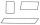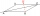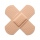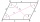# The area of rhomboid problems

#### Number of problems found: 12

• RhomboidCalculate the circumference and area of the rhomboid with sides 20 and 14, with their angle 50°.
• ParallelogramRhomboid (parallelogram) has a longer side of 50 cm long. The size of its one height is four times the size of its second height. Calculate the length of the shorter side of this rhomboid in the centimeters.
• Tetrahedral prism - rhomboid baseCalculate the area and volume tetrahedral prism that has base rhomboid shape and its dimensions are: a = 12 cm, b = 70 mm, v_a = 6 cm, v_h = 1 dm.
• PrismCalculate the surface area and volume of a prism with a body height h = 10 cm and its base has shape of a rhomboid with sides a = 5.8 cm, b = 3 cm and the distance of its two longer sides is w = 2.4 cm.
• Tetrahedral prismCalculate surface and volume tetrahedral prism, which has a rhomboid-shaped base, and its dimensions are: a = 12 cm, b = 7 cm, ha = 6 cm and prism height h = 10 cm.
• ParallelogramCalculate area of the parallelogram ABCD as shown if |AB| = 19 cm, |BC| = 18 cm and angle BAD = 90°
• Trapezoid MO-5-Z8ABCD is a trapezoid that lime segment CE is divided into a triangle and parallelogram, as shown. Point F is the midpoint of CE, DF line passes through the center of the segment BE, and the area of the triangle CDE is 3 cm2. Determine the area of the trape
• Two patchesPeter taped the wound with two rectangular patches (one over the other to form the letter X). The area sealed with both patches at the same time had a content of 40cm2 and a circumference of 30cm. One of the patches was 8cm wide. What was the width of the
• RhumblineFind circumference and area of the rhumbline ABCD if the short side AD of which has a length of 5 cm, and the heel of the height from D leading to the AB side divides the AB side into two sections of 3 cm and 4 cm.
• ParallerogramIn parallelogram ABCD is a = 2.5 cm and height to side a is 7.2 cm and height to side b is 4 cm. Find the side length b.
• Dusana) Dusan break two same window, which has triangular shape with a length of 0.8 m and corresponding height 9.5 dm. Find how many dm2 of glass he needs to buy for glazing of these windows. b) Since the money to fix Dusan has not, must go to the paint job
• Black buildingKeith built building with a rectangular shape 6.5 m × 3.9 m. Calculate how much percent exceeded the limit 25 m2 for small building. Building not built in accordance with the law is called "black building". Calculate the angle that the walls were clenchi

We apologize, but in this category are not a lot of examples.
Do you have an interesting mathematical word problem that you can't solve it? Submit a math problem, and we can try to solve it.

We will send a solution to your e-mail address. Solved examples are also published here. Please enter the e-mail correctly and check whether you don't have a full mailbox.

Please do not submit problems from current active competitions such as Mathematical Olympiad, correspondence seminars etc...

Examples of area of plane shapes. Rhomboid Problems.